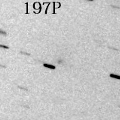# 197P/LINEAR (2013)###Pictures###Orbital Elements

```Epoch 2013 Mar. 9.0 TT = JDT 2456360.5
T 2013 Mar. 24.85318 TT                                 Kobayashi
q   1.0614595            (2000.0)            P               Q
n   0.20304089     Peri.  188.74084     -0.27022237     +0.87800162
a   2.8669077      Node    66.38995     -0.85523774     -0.03042095
e   0.6297546      Incl.   25.54241     -0.44220842     -0.47768998
P   4.85
From 304 observations 2003 May 23-2013 Sept. 25, mean residual 0".7.
Nongravitational parameters A1 = +0.00, A2 = +0.0018.
```

###Magnitudes Graph

```        m1 = 17.5 + 5 log d + 10.0 log r           [   , 20]  (             - 2013 Apr. 13)
m1 =  8.0 + 5 log d + 30   log r(t + 100)  [ 20,106]  (2013 Apr. 13 - 2013 July  8)
m1 = 17.5 + 5 log d + 10.0 log r           [106,   ]  (2013 July  8 -             )
```##### The orbital elements are published on M.P.E.C. 2015-M77. The magnitudes graphs are made with Comet for Windows.